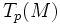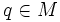# Hopf-Rinow theorem

(diff) ← Older revision | Latest revision (diff) | Newer revision → (diff)

## Statement

Let$(M,g)$ be a Riemannian manifold (viz$M$ is a differential manifold equipped with a Riemannian metric$g$). Then the Hopf-Rinow theorem states the following equivalent things:

1. If$M$ is geodesically complete, then given any two points$p,q \in M$ there is a minimizing geodesic joining$p$ and$q$.
2. For any point$p \in M$, the exponential map at$p$ is surjective from$T_p(M)$ to$M$, and any length-minimizing curve from$p$ to$q$ for some$q \in M$, occurs as the image of a straight line from the origin, under the exponential map.

## Proof

• Given$p \in M$, there exists$\epsilon > 0$, such that the exponential map takes the unit sphere of radius$\epsilon$ about$p$ to the set of points on the manifold at distance$\epsilon$ from$p$, with the minimal geodesics from$p$ being exponentials of the radial paths.

The upshot of this is: given$p \in M$, there is an$\epsilon$ such that the set of points at distance$\epsilon$ from$p$ is a compact set, and such that there is a unique minimizing geodesic from$p$ to each of them

• Any geodesic can be extended indefinitely (and uniquely) in both directions (this follows from the assumption that our manifold is geodesically complete)

The proof beyond these two facts is purely geometric (that is, it only uses standard properties of metrics and continuous maps).